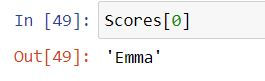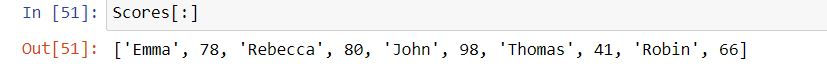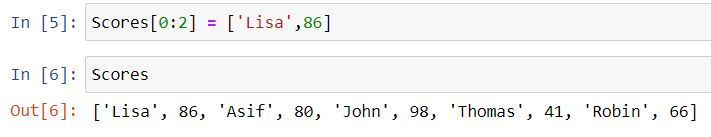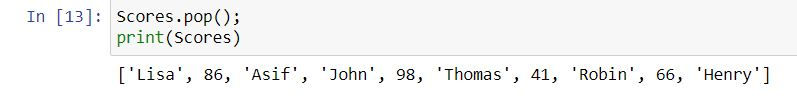top of page
Search
• Ekta Aggarwal

# Lists

Lists are a data object in Python which can store multiple values and the elements need not be of same data type.

In this tutorial we shall learn about lists in great detail!

### Characteristics of a list

1. A list can have multiple elements: Let us create a list containing the scores of the students. A list is created in [ ] square brackets.

```Scores = [78,80,98,41,66]
type(Scores)```2. Lists can have elements of multiple data types: Following is our list of scores - which contains student names (strings) and their scores (numeric)

`Scores = ['Emma',78,'Rebecca',80,'John',98,'Thomas',41,'Robin',66]`

3. A list can contain several lists! Following list contains scores of students where each element is itself a list!

`list2 = [['Emma',78],['Rebecca',80],['John',98],['Thomas',41],['Robin',66]]`

## Subsetting lists

Understanding with the help of an example!

`Scores = ['Emma',78,'Rebecca',80,'John',98,'Thomas',41,'Robin',66]`

Python indexing begins from 0. Thus to get the first element of the list we write:

list_name . Square brackets denote that we want to extract an element from our list.

`Scores`Following code will return fourth element (indexing starts from 0, thus 3 represents 4th index)

`Scores`Writing a semi-colon : in square brackets [ ] in front of the list name would return all the elements.

`Scores[:]`In Python an index of -1 indicates extract the last element of our list!

`Scores[-1]`Similarly an index of -2 indicates extract the second last element

`Scores[-2]`## List Slicing

Extracting multiple elements of a list is called list slicing (you are slicing down i.e. cutting down your list)

`Scores[1:4] # Extract the elements from first index to third index (index 4 is excluded) i.e. positions 2 to 4. (Remember Python indexing starts from 0)`If we write [N: ] in front of a list then it tells Python to extract all the elements starting from Nth index.

`Scores[1:]`If we write [:N] in front of a list then it tells Python to extract elements from the starting till the (N-1)th index (N is excluded!)

`Scores[:4]`## Index function

Let us again create our list:

`Scores = ['Emma',78,'Rebecca',80,'John',98,'Thomas',41,'Robin',66]`

index( ) function returns the Python index for the list element defined in the parenthesis. In our list John appears at 4th index!

`Scores.index('John')`
`Scores.index(80)`## List manipulation

List manipulation involves:

-Changing the elements

-Removing elements from a list

`Scores = ['Emma',78,'Rebecca',80,'John',98,'Thomas',41,'Robin',66]`

### Changing the elements

By defining list_name[index] = 'New_value' we can update the values in our list

```Scores = 'Asif'
Scores```We can also change multiple elements of a list in one command. In the following code we are updating 0th and 1st index values as 'Lisa' and 86

```Scores[0:2] = ['Lisa',86]
Scores```We can also append or add elements in our list.

Method 1: Using a + symbol we can add elements to our list.

In the following code we are adding a list ['Henry',92] at the end of our original list Scores.

```Scores = Scores + ['Henry',92];
print(Scores)```Method 2: Using .append( ) command

In the following code we are adding an element "Bella" at the end of our list Scores.

```Scores.append('Bella');
print(Scores)```### Removing elements from a list

We can remove elements from a list using .remove( ) command:

```Scores.remove('Bella');
print(Scores)```We can also insert elements by specifying the index using insert ( ) function. In the following code we have added Bella at 2nd index (i.e. 3rd position) in our list.

```Scores.insert(2,'Bella');
print(Scores)```Let us remove this element for the sake of simplicity.

```Scores.remove('Bella');
print(Scores)```

We can also remove the elements by specifying the index using pop( ) command. In the code below we are deleting the element at 3rd index i.e. 4th position.

```Scores.pop(3);
print(Scores)```If you do not specify anything in the pop( ) command then by default it removes the last element from the list.

```Scores.pop();
print(Scores)```## Sorting a list!

Let us create our list again!

`Scores = [78,80,98,41,66]`

We can sort the elements of a list using sort( ) command. By default Python sorts by ascending order.

```Scores.sort();
Scores```

To sort the list elements in descending order we define reverse = True inside sort( ) function.

```Scores.sort(reverse= True);
print(Scores)```## Counting

We can count the number of occurrence of an element using count( ) function.

```Scores = ['Emma',78,'Rebecca',80,'John',98,'Thomas',41,'Robin',66]
Scores.append('John');
print(Scores)
Scores.count('John')```

In our list John is appearing twice!bottom of page